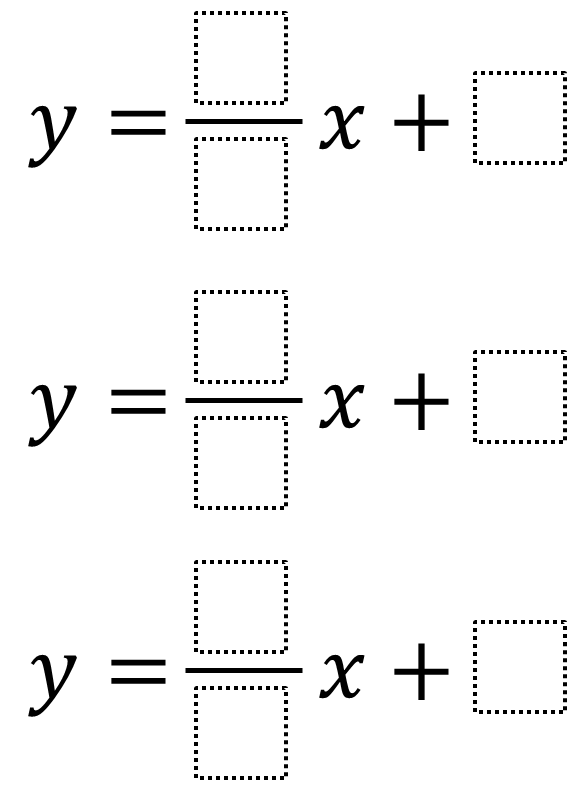# Systems of Equations – One Solution

Directions: Using the integers from -9 to 9, at most one time each, create a system of three-equations such that the solution is (1,1).### Hint

How can we determine whether a line goes through the point (1, 1)?

One answer is y = (0/9)x + 1,  y = (-4/2)x + 3, and y= (-6/-1)x + -5.

Source: Audrey Mendivil, Daniel Luevanos, and Robert Kaplinsky

### Check Also

Directions: Directions: Using the integers from −9 to 9 at most once each, place one …

### One comment

1.That’s too easy!
I think i’m correct, but probably wrong if I didn’t follow the rules.
I think that the solution is;
1. y=x
2. y=-x+2
3. y=(0/n*)x +1

n* means every integer from -9 to 9, as it has been said on the rules (that is 0, obviously)